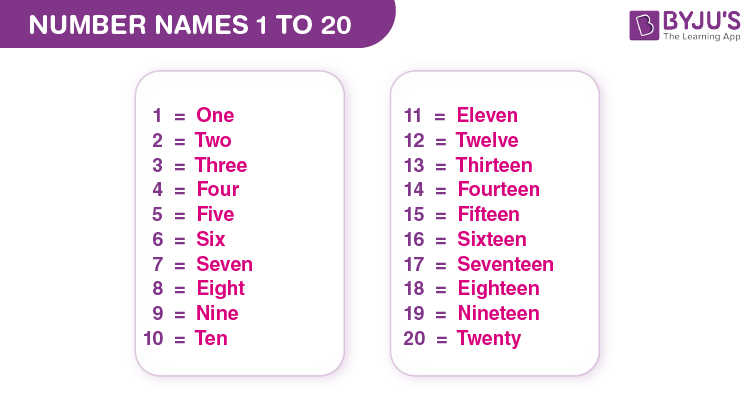Checkout JEE MAINS 2022 Question Paper Analysis : Checkout JEE MAINS 2022 Question Paper Analysis :

# Number Names 1 to 20

Number names 1 to 20 in Maths represent numbers 1 to 20 in word format. For example, the number 7 is written as seven, and the number 11 is written as eleven, and so on. The number names from 1 to 20 are written using place values such as ones, tens, hundreds, etc. Here, we will learn the number names from 1 to 20, the rules for writing number names from 1 to 20, and various solved problems.

## Number Names 1 to 20 Chart

Number names 1 to 20 chart is provided here for kids to learn 1 to 20 spelling in English.## Numbers from 1 to 20 in Words

Number names from 1 to 20 are listed in tabular form. Look at the table below to quickly learn the number names from 1 to 20.

 Numbers from 1 to 20 Number Names 1 to 20 1 One 2 Two 3 Three 4 Four 5 Five 6 Six 7 Seven 8 Eight 9 Nine 10 Ten 11 Eleven 12 Twelve 13 Thirteen 14 Fourteen 15 Fifteen 16 Sixteen 17 Seventeen 18 Eighteen 19 Nineteen 20 Twenty

## Rules to Write Number Names 1 to 20

The rules for writing numbers in words from 1 to 20 are as follows:

• To begin, learn the names of the numbers from 1 to 9. i.e., One, two, three, four, five, six, seven, eight, and nine.
• Learn the names of the numbers 10 and 11. i.e., ten and eleven.
• To write the number from 12 to 19, add the suffix “teen” to the numbers in words from two to nine. For example, the number 16 is written as sixteen. i.e., six+teen = sixteen.
• Finally, for the number 20, add the suffix “ty” to the number name. i.e., 20 = twenty [Note: for counts in tens from 20 to 90, the number names always end in “ty”].

### Number Names 1 to 20 Examples

Example 1:

Write the number name for the following numbers:

1. 6
2. 11
3. 19

Solution:

The number names are:

1. 6 – Six
2. 11 – Eleven
3. 19 – Nineteen.

Example 2:

What is the value of eight plus four?

Solution:

Eight = 8

Four = 4

Eight plus four = 8+4 = 12

So, 12 in words is twelve

Therefore, the value of eight plus four is twelve.

### Number Names 1 to 20 Worksheet

1. Write the number names for the following numbers:

(a) 4 = ____

(b) 10 = ____

(c) 14 = ____

2. What is the value of nineteen minus nine?

## Frequently Asked Questions on Number Names 1 to 20

### What is meant by the number names 1 to 20 in Maths?

The number names 1 to 20 represent the numeral 1 to 20 in word format.

### Write the number names from 1 to 20.

The number names from 1 to 20 are as follows: 1 – one, 2 – two, 3 – three, 4 – four, 5 – five, 6 – six, 7 – seven, 8 – eight, 9 – nine, 10 – ten, 11 – eleven, 12 – twelve, 13 – thirteen, 14 – fourteen, 15 – fifteen, 16 – sixteen, 17 – seventeen, 18 – eighteen, 19 – nineteen, 20 – twenty.

### Express the value of 10+9 in words.

The value of 10+9 is 19. Thus, the number 19 in words is nineteen.